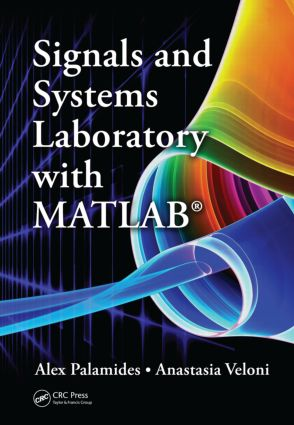Signals and Systems Laboratory with MATLAB

1st Edition

CRC Press

567 pages | 704 B/W Illus.

Purchasing Options:\$ = USD
Hardback: 9781439830550
pub: 2010-08-13
SAVE ~\$22.00
\$110.00
\$88.00
x
eBook (VitalSource) : 9780429065644
pub: 2010-08-13
from \$55.00

FREE Standard Shipping!

Description

With its exhaustive coverage of relevant theory, Signals and Systems Laboratory with MATLAB® is a powerful resource that provides simple, detailed instructions on how to apply computer methods to signals and systems analysis. Written for laboratory work in a course on signals and systems, this book presents a corresponding MATLAB implementation for each theoretical concept introduced, making it a powerful learning tool for engineers, scientists, and students alike.

MATLAB code is used in problems and examples presented throughout the book. This code and other learning materials are available in a downloadable supplement.

Due to the extensive—and truly unique—integration of MATLAB throughout this book, the authors provide a complete tutorial on use of the language for signals and systems analysis. With more than 5,000 lines of MATLAB code and more than 700 figures embedded in the text, the material teaches readers how to program in MATLAB and study signals and systems concepts at the same time, giving them the tools to harness the power of computers to quickly assess problems and then visualize their solutions.

Among its many useful features, this book:

• Offers complete coverage of the signals and systems theory, starting with elementary signals and concluding with state-space modeling
• Contains more than 400 examples and chapter-end solved problems
• Executes commands one-by-one at the MATLAB command prompt, and results, along with comments, encouraging students to learn MATLAB on the fly

• A detailed MATLAB tutorial to introduce a beginner programmer to the language
• Laboratory exercises that give students hands-on experience and help professors organize a course laboratory component
• Presentation of continuous- and discrete-time in parallel fashion, effectively illustrating the similarities and differences between the two
• Step-by-step examples that present data in tabular format and usually offer several different solutions to each problem

Introduction to MATLAB®

Working Environment

Getting Started

Memory Management

Vectors

Matrices

Plotting with MATLAB

Complex Numbers

M-Files

Input-Output Commands

File Management

Logical-Relational Operators

Control Flow

Symbolic Variables

Polynomials

(Pseudo)Random Numbers

Signals

Categorization by the Variable Type

Basic Continuous-Time Signals

Discrete-Time Signals

Properties of Signals

Transformations of the Time Variable for Continuous-Time Signals

Transformations of the Time Variable for Discrete-Time Signals

Systems

Systems Classification

Properties of Systems

Time Domain System Analysis

Impulse Response

Continuous Time Convolution

Convolution Properties

Interconnections of Systems

Stability

Discrete-Time Convolution

Systems Described by Difference Equations

Filters

Stability Criterion for Discrete-Time Systems

Systems Described by Differential Equations

Step Response of a System

Fourier Series

Orthogonality of Complex Exponential Signals

Complex Exponential Fourier Series

Trigonometric Fourier Series

Fourier Series in the Cosine with Phase Form

Plotting the Fourier Series Coefficients

Fourier Series of Complex Signals

Fourier Series of Periodic Signals

Line Spectra

Properties of Fourier Series

Symmetry

Parseval’s Identity

Criterion for the Approximation of a Signal by a Fourier Series Expansion

Relationship between Complex Exponential and Trigonometric Fourier Series Coefficients

Fourier Transform

Mathematical Definition

The Commands fourier and fourier

Fourier Transform Pairs

Properties of Fourier Transform

Convolution in Time and Frequency

Symmetry of the Real and Imaginary Parts of Fourier Transform

Parseval’s Theorem

Autocorrelation and Cross-Correlation

Fourier Analysis of Discrete-Time Signals

Discrete-Time Fourier Transform

Properties of Discrete-Time Fourier Transform

Parseval’s Theorem for Discrete-Time Fourier Transform

Discrete Fourier Transform

Properties of Discrete Fourier Transform

Inverse Discrete Fourier Transform

Circular Shift of a Sequence

Circular Convolution

Fast Fourier Transform

Relationship between DFT and DTFT

Relationship between Fourier Transform and Discrete Fourier Transform

Linear Convolution Computation via Fast Fourier Transform

Frequency Response

Continuous-Time Frequency Response

The Command freqs

The Command lsim

System Response to Sinusoidal Input

Ideal Filters

Frequency Response of Discrete-Time Systems

The Command freqz

System Response to Discrete-Time Sinusoidal Input

Moving Average Filter

The Laplace Transform

Mathematical Definition

Commands laplace and ilaplace

Region of Convergence

Laplace Transform Pairs

Laplace Transform Properties and Theorems

Partial Fraction Expansion of a Rational Function

Convolution in Time and in Complex Frequency

Using the Laplace Transform to Solve Differential Equations

z-Transform

Mathematical Definition

Commands ztrans and iztrans

Region of Convergence

z-Transform Pairs

Properties of z-Transform

Partial Fraction Expansion of a Rational Function

Using the z-Transform to Solve Difference Equations

Transfer Function

Continuous-Time Systems

The tf Command

Stability of Continuous-Time Systems

Transfer Function in Zero-Pole-Gain Form

Interconnections of Systems

Continuous-Time System Response

Discrete-Time Systems

The Command tf for Discrete-Time Systems

Stability of Discrete-Time Systems

Discrete-Time System Response

Conversion between Continuous-Time and Discrete-Time Systems

Transfer Function and Frequency Response

Bode Plot

State-Space Representation

Suggested Laboratory Exercises

Introduction to MATLAB

Signals

Systems

Time Domain System Analysis

Fourier Series

Fourier Transform

Fourier Analysis of Discrete-Time Systems

Frequency Response

Laplace Transform

z-Transform

Transfer Function

Appendices

References

Index

Dr. Alex Palamides is with the European Space Agency, European Space Research and Technology Centre, Noordwijk, the Netherlands. He is the author/coauthor of several research contributions published in journals and for conferences, and he has authored one other textbook. His research interests lie in the areas of signal processing, dynamic systems, telecommunications, and differential equations.

Professor Anastasia Veloni is with Technological Educational Institute of Piraeus, Department of Electronic Computer Systems, Athens, Greece. She has extensive educational experience in a variety of courses in the field of signals and systems. She is the author/coauthor of three other textbooks. Her research interests lie in the areas of signal processing, dynamic systems, and automatic control.